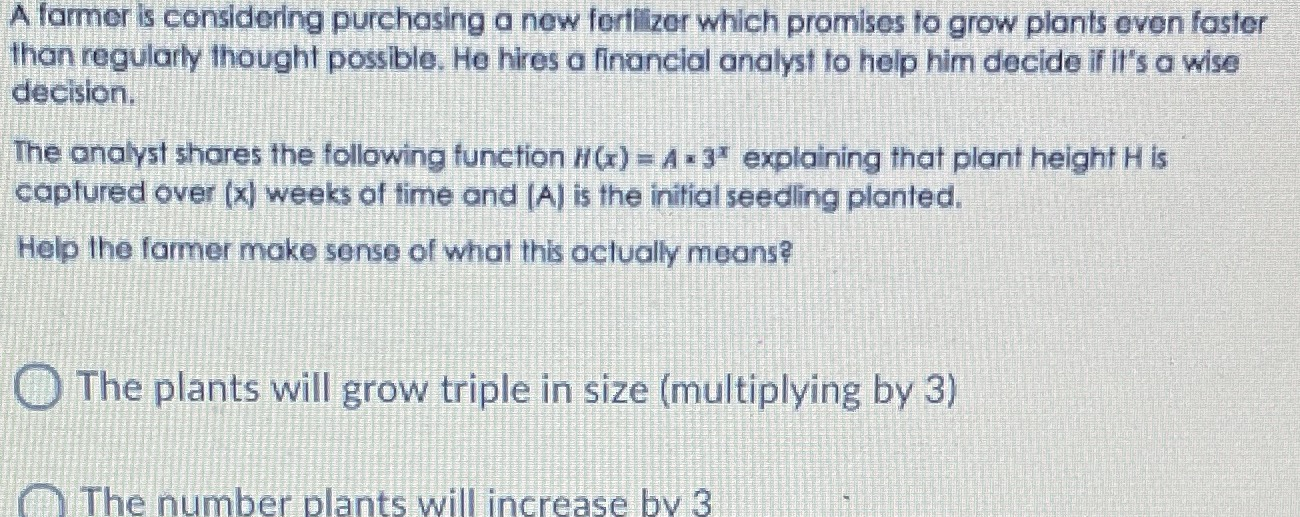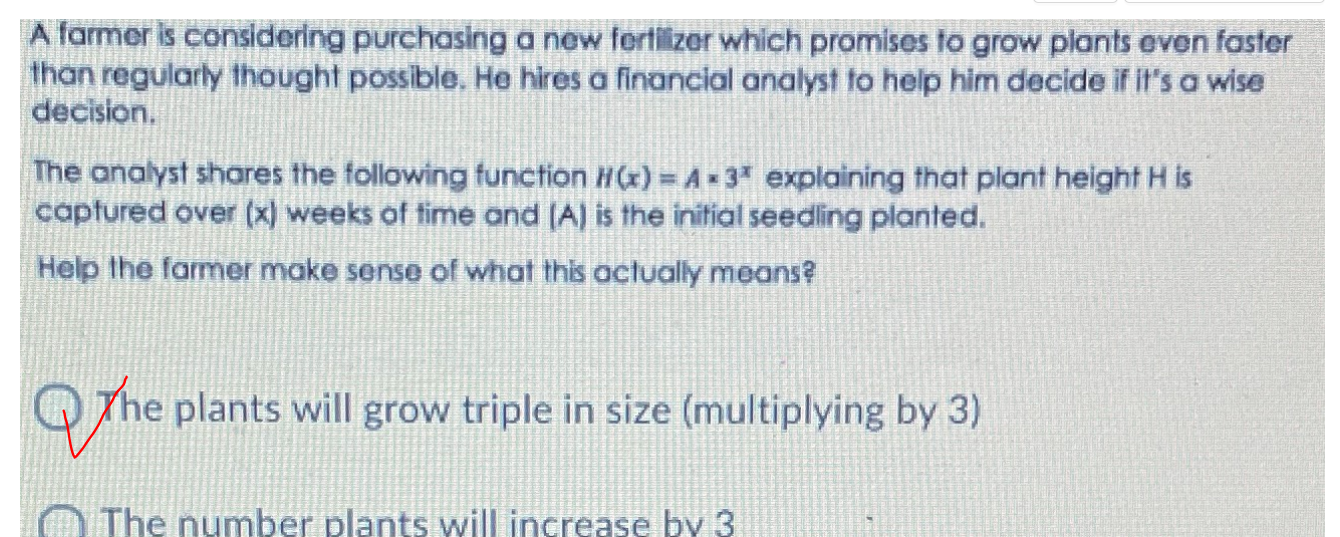### ¿Todavía tienes preguntas de matemáticas?

Pregunte a nuestros tutores expertos
Algebra
PreguntaA former is considering purchasing a new fertizer which promises to grow plonits even foster than regularly thought possible. He hires a financial onalyst to help him decide if it's o wise decision. The onalyst shores the following function $$H ( x ) = A = 3 ^ { x }$$ explaining that plant height $$H$$ is coptured over $$( x )$$ weeks of time ond $$( A )$$ is the initiol seeding planted. Help the farmer make sense of what this oclually means? The plants will grow triple in size (multiplying by $$3$$ ) The number plants will increase bv $$3$$